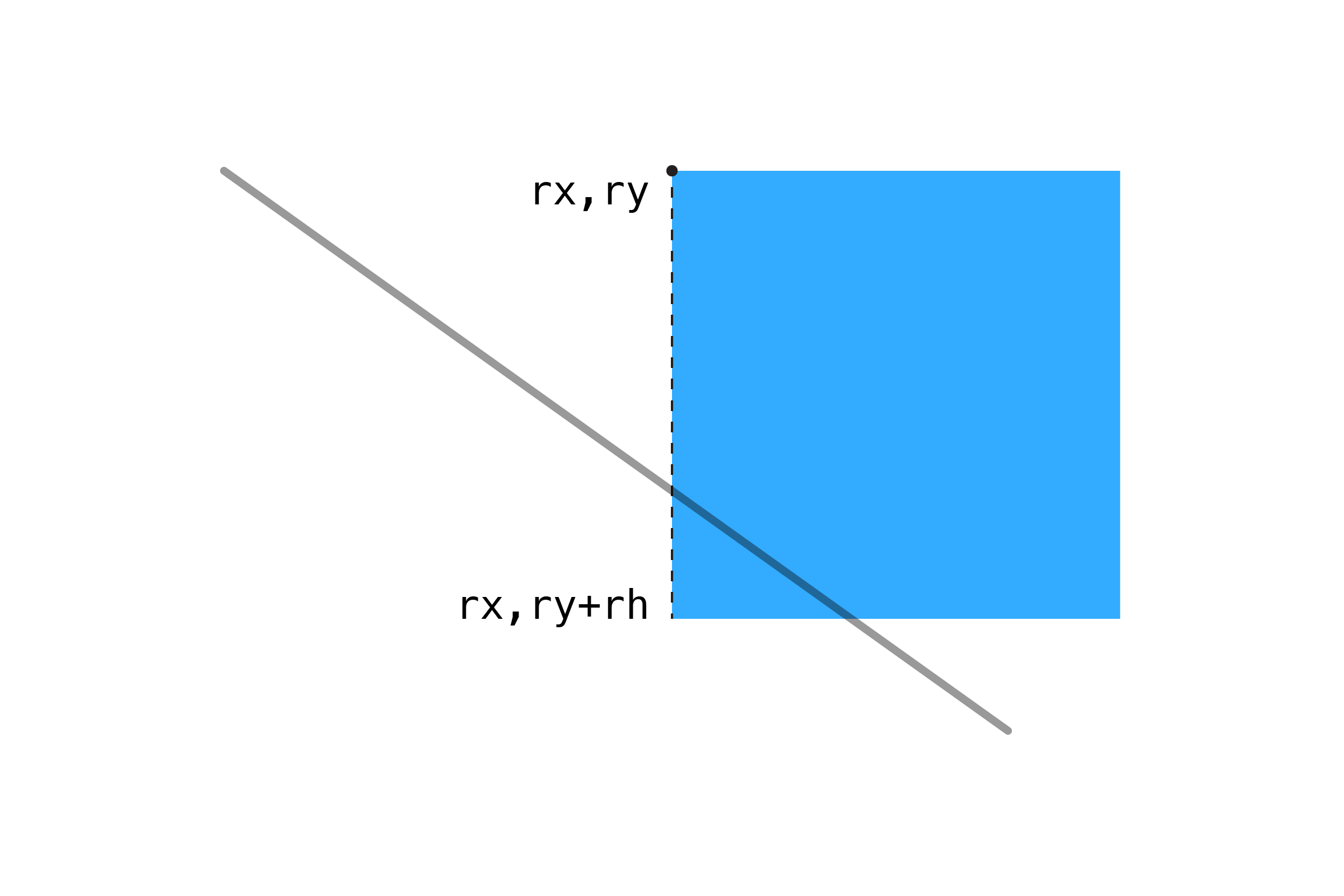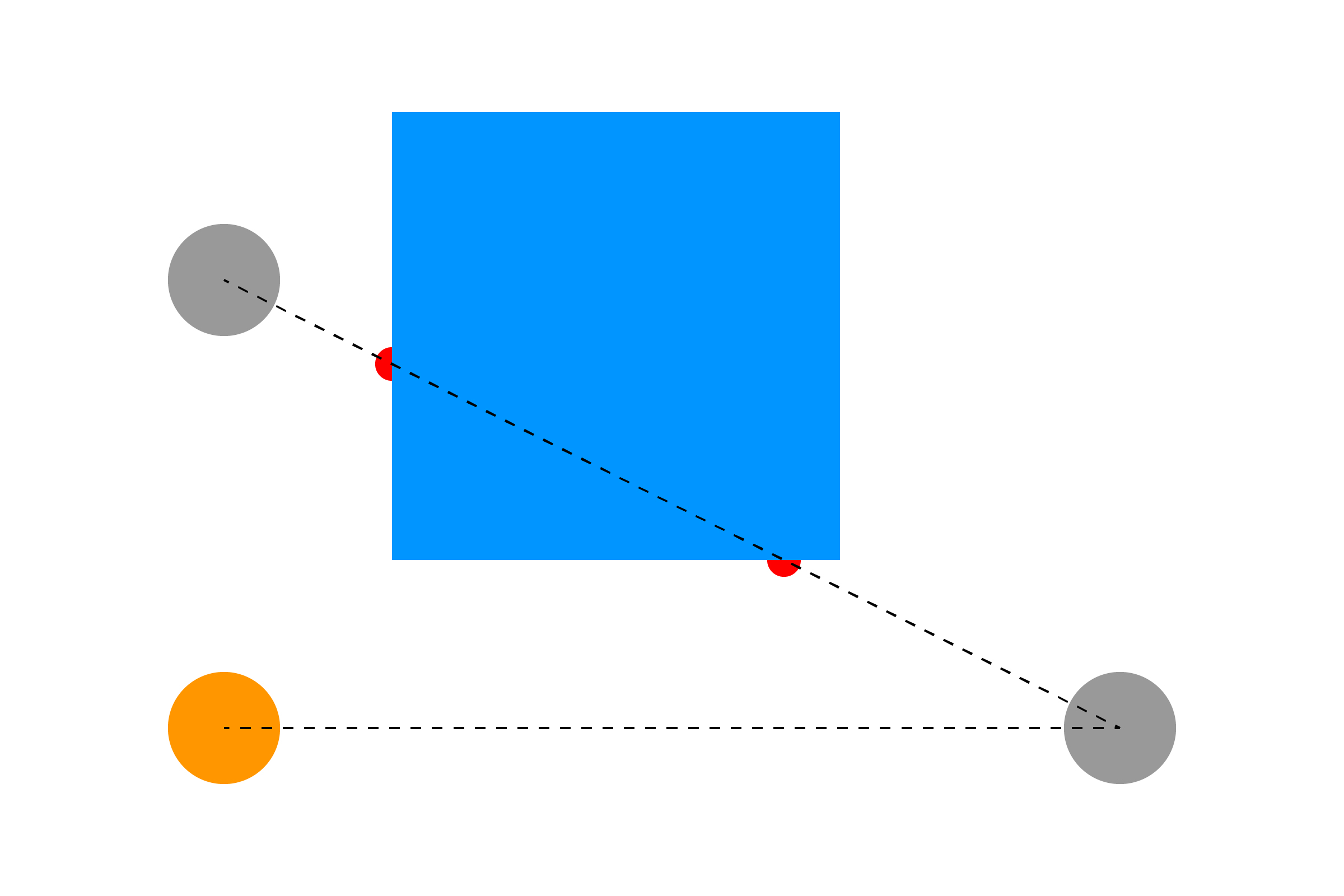[ your browser does not support the canvas tag ]

# LINE/RECTANGLE

We've actually already covered how to check if a line has hit a rectangle: it's really just four Line/Line collisions, one for each side!

For example, the left edge of the square starts at `(rx,ry)` and extends down to `ry+rh`. We can treat that as a line, using the algorithm we made in the last section:

``````boolean left =   lineLine(x1,y1,x2,y2, rx,ry, rx,ry+rh);
``````

This can be more easily visualized like this:We do the same for the other three sides:

``````boolean left =   lineLine(x1,y1,x2,y2, rx,ry,rx, ry+rh);
boolean right =  lineLine(x1,y1,x2,y2, rx+rw,ry, rx+rw,ry+rh);
boolean top =    lineLine(x1,y1,x2,y2, rx,ry, rx+rw,ry);
boolean bottom = lineLine(x1,y1,x2,y2, rx,ry+rh, rx+rw,ry+rh);
``````

If any of the above statements are true, the line has hit the rectangle.

``````if (left || right || top || bottom) {
return true;
}
return false;
``````

A full example is below. Note that the red dots are drawn in the Line/Line function, showing where the line intersects the rectangle. You can delete them from the function if you don't want them in your finished project.

``````float x1 = 0;      // points for line (controlled by mouse)
float y1 = 0;
float x2 = 0;      // static point
float y2 = 0;

float sx = 200;    // square position
float sy = 100;
float sw = 200;    // and size
float sh = 200;

void setup() {
size(600, 400);

strokeWeight(5);  // make the line easier to see
}

void draw() {
background(255);

// set end of line to mouse coordinates
x1 = mouseX;
y1 = mouseY;

// check if line has hit the square
// if so, change the fill color
boolean hit = lineRect(x1,y1,x2,y2, sx,sy,sw,sh);
if (hit) fill(255,150,0);
else fill(0,150,255);
noStroke();
rect(sx, sy, sw, sh);

// draw the line
stroke(0, 150);
line(x1, y1, x2, y2);
}

// LINE/RECTANGLE
boolean lineRect(float x1, float y1, float x2, float y2, float rx, float ry, float rw, float rh) {

// check if the line has hit any of the rectangle's sides
// uses the Line/Line function below
boolean left =   lineLine(x1,y1,x2,y2, rx,ry,rx, ry+rh);
boolean right =  lineLine(x1,y1,x2,y2, rx+rw,ry, rx+rw,ry+rh);
boolean top =    lineLine(x1,y1,x2,y2, rx,ry, rx+rw,ry);
boolean bottom = lineLine(x1,y1,x2,y2, rx,ry+rh, rx+rw,ry+rh);

// if ANY of the above are true, the line
// has hit the rectangle
if (left || right || top || bottom) {
return true;
}
return false;
}

// LINE/LINE
boolean lineLine(float x1, float y1, float x2, float y2, float x3, float y3, float x4, float y4) {

// calculate the direction of the lines
float uA = ((x4-x3)*(y1-y3) - (y4-y3)*(x1-x3)) / ((y4-y3)*(x2-x1) - (x4-x3)*(y2-y1));
float uB = ((x2-x1)*(y1-y3) - (y2-y1)*(x1-x3)) / ((y4-y3)*(x2-x1) - (x4-x3)*(y2-y1));

// if uA and uB are between 0-1, lines are colliding
if (uA >= 0 && uA <= 1 && uB >= 0 && uB <= 1) {

// optionally, draw a circle where the lines meet
float intersectionX = x1 + (uA * (x2-x1));
float intersectionY = y1 + (uA * (y2-y1));
fill(255,0,0);
noStroke();
ellipse(intersectionX, intersectionY, 20, 20);

return true;
}
return false;
}
``````

This algorithm can also be used to test line-of-sight. Let's say you have two objects and a rectangular obstacle: if you draw a line between one object and another, then check if it has hit the rectangle, you can tell if the objects can "see" each other or if they are hidden behind the obstacle.For an example of this in code, see the Line Of Sight example in my Processing teaching repository.

NEXT: Section 3 Challenges